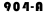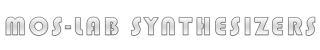FUNCTION DESCRIPTION :

The 904A Voltage-Controlled Low Pass Filter attenuates frequencies above the fixed control voltage cutoff point at a rate of 24 dB per octave. The cutoff frequency is voltage controlled through the control input jacks. The sum of the applied control voltages doubles the frequency of the cutoff point for each one Volt increase. The regeneration potentiometer varies the amount of internal feedback, creating a resonant peak at the cutoff frequency. This resonant peak will break into oscillation at clockwise settings of the regeneration potentiometer, creating a voltage controlled sine wave generator. The fixed control voltage potentiometer covers a 12 Volt (octave) range. The overall range of the Frequency potentiometer is determined by the Frequency Range switch, which moves the frequency cutoff range in two-octave steps.

TECHNICAL SPECIFICATION :

Signal Input Level: 0 dBm nominal, +10dBm max without clipping

Signal Input Impedance : 9,2kΩ

Control Input Impedance :100kΩ

Output Impedance : 680Ω (AC coupled)

Cutoff Frequency fc range : 1Hz to 80 kHz

Attenuation slope above Fc : 24 dB/octave

Control Input Frequency Response : DC to 50 kHz

Number of control inputs : 3

Power supply requirements : +12V@70mA / -12@40mA